Definition Of Hyperbola

Hyperbola is a conic section in which difference of distances of all the points from two fixed points (called foci) is constant.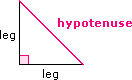The general equation for hyperbola is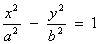.
The eccentricity (e) of a hyperbola is always greater than 1, e > 1.
The slope of asymptotes for both horizontal and vertical hyperbola is.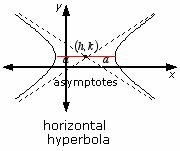The equation for the horizontal hyperbola is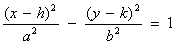.

Video Examples: Hyperbola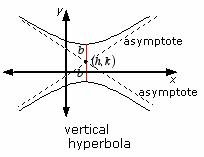The equation for the vertical hyperbola is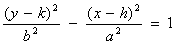.
The foci of the hyperbola are (± ; c, 0).

Example of Hyperbola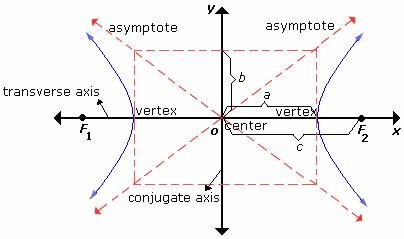Solved Example on Hyperbola

Ques: Choose the standard form of the equation for the hyperbola shown.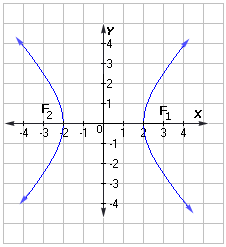Choices: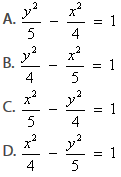Step 5: Since the transverse axis is horizontal, the standard form of the hyperbola isor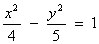.